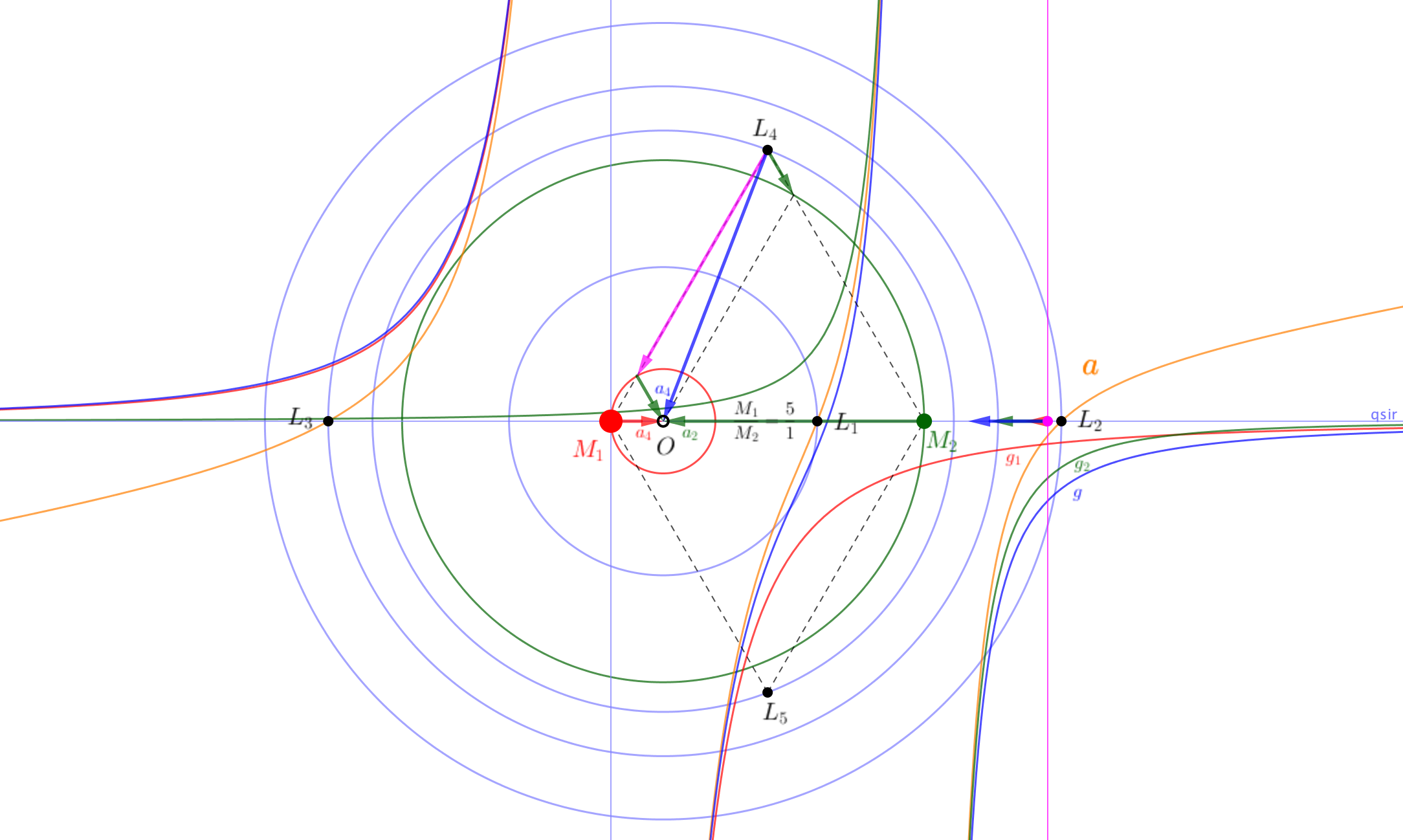17

## the Lagrange points$\frac{GM_1M_2}{R^2}=M_1\omega ^2 R_1$$\frac{GM_1M_2}{R^2}=M_2\omega ^2 R_2$
$\frac{R_1}{R_2}=\frac{M_2}{M_1}$$\omega^2=\frac{G(M_1+M_2)}{R^3}$

### On this day..